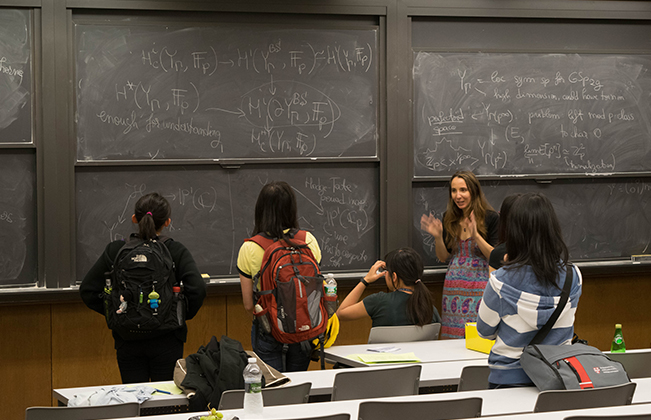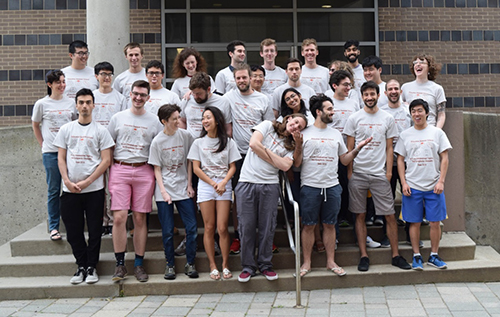# Summer School in Geometric Analysis – June 2019A three-week intensive program at Princeton University for 25 advanced undergraduates and first-year graduate students consisting of courses in geometric analysis.  Geometric analysis is the branch of mathematics which uses methods in analysis and PDE to study problems in geometry. The goal of the program is to introduce some basic tools in analysis and explore their connections to geometric analysis. Topics will include surface theory, minimal and constant mean curvature surfaces, min-max methods, Allen-Cahn equations, zero sets of harmonic functions and eigen-functions, Sobolev inequalities, problem of prescribing curvatures, holography in conformal geometry and surface theory in 3D Heisenberg.

There will be eight courses, each with four lectures and TA review sessions. In addition, there will be a three-day mini-conference to cover recent research topics in geometric analysis as well as a special one-day conference Diversity in Geometry Festival associated with the program.

Week 1 (June 10-14, 2019)

Introduction to minimal and constant mean curvature surfaces in 3-manifolds
Instructor: Ana Menezes

In this course, we will give some introduction to the study of minimal and constant mean curvature surfaces in 3-manifolds, giving special attention to those immersed in a simply-connected homogeneous space. We will talk about some classical theorems as well as some recent achievements.

Extremal metrics for some variational geometric quantities
Instructor: Rafael Montezuma

This mini-course concerns the existence and characterization of Riemannian metrics that are extremal for some geometric invariants related to either the lengths of geodesics, areas of minimal surfaces, or eigenvalues and eigenfunctions of an operator (such as the Laplacian, or the Dirichlet-to-Neumann operators). Towards the end, I will discuss some recent joint work with Lucas Ambrozio that concerns extremal metrics for the min-max width of Riemannian 3-spheres, and the 2-systole of 3-dimensional real projective spaces. The min-max width is an invariant of the metric, which is closely related to the existence theory of minimal surfaces in general closed Riemannian manifolds; it is a particular critical value of the area functional.

Min-max theory for the area functional and applications
Instructor: Fernando Marques

These lectures concern the existence theory of closed minimal hypersurfaces in closed Riemannian manifolds of dimension at least three. These hypersurfaces are critical points for the area functional, and hence their study can be seen as a high-dimensional generalization of the classical theory of closed geodesics (Birkhoff, Morse, Lusternik, Schnirelmann, et al.). The best result until very recently, due to Almgren' 65, Pitts' 81 and Schoen-Simon'81, was the existence of at least one closed minimal hypersurface in every closed Riemannian manifold. We will discuss the methods I have developed with Andre Neves, for the past few years, to approach this problem through the variational point of view. These ideas have culminated with the discovery that minimal hypersurfaces in fact abound.

Week 2 (June 17-21, 2019)

Geometric features of the Allen-Cahn equation
Instructor: Otis Chodish

The Allen--Cahn equation models the interaction between disparate phases of matter. Surprisingly, it is also linked to minimal surfaces and mean curvature flow. We will discuss these links and other features, with an eye towards geometric applications. Knowledge of basic measure theory, elliptic PDE, and Riemannian geometry would be useful but not strictly necessary.

Zeros of harmonic functions and Laplace eigenfunctions
Instructor: Aleksandr Logunov

We will discuss geometrical and analytic properties of zero sets of harmonic functions and eigenfunctions of the Laplace operator. For harmonic functions on the plane there is an interesting relation between local length of the zero set and the growth of harmonic functions. The larger the zero set is, the faster the growth of harmonic function should be and vice versa. A curious object is Laplace eigenfunctions on two dimensional sphere, which are restrictions of homogeneous harmonic polynomials of three variables onto the sphere. They are called spherical harmonics. Zero sets of such functions are unions of smooth curves with equiangular intersections. Topology of zero set could be quite complicated, but the total length of the zero set of any spherical harmonic of degree n is comparable to n. Though the Laplace eigenfunctions are known for ages, we still don't understand them well enough (even the spherical harmonics). A couple of open problems will be formulated along the course.

Week 3 (June 24-28, 2019)

Sobolev inequalities and the problem of prescribing curvatures
Instructor: Alice Chang

We will start with the derivation of the classical Sobolev inequalities on domains in the Euclidean space. We then establish the connection of these inequalities to the problem of "prescribing curvatures" on manifolds in conformal geometry. On manifolds of dimension bigger than two, a model case is the "Yamabe problem" of prescribing constant scalar curvature. On compact surfaces, e.g. on the two sphere, the problem of prescribing Gaussian curvature is the famous "Nirenberg problem", where a borderline Sobolev inequality plays an important role.

We will also touch on the case of prescribing a class of higher order curvature polynomials on manifolds of dimension 4.

Holography in conformal geometry
Instructor: Stephen McKeown

One of the approaches to studying conformal geometry is to view a conformal space as the "boundary at infinity" of an Einstein space of one dimension higher. There are surprising and deep connections between the two geometries. We will describe what the above words mean and study some of the basic geometric constructions in this setting.

Geometry of surfaces in the 3-dimensional Heinsenberg
Instructor: Paul Yang

We will introduce the Heisenberg geometry as the flat model of 3-dimensional CR geometry. After a brief discussion of the Webster connection and curvature, we will discuss the isoperimetric problem to minimize the surface area that bounds a domain of fixed volume as well as the analogue of the Willmore problem for this geometry.Participants of the 2018 Princeton Summer School in Topology and Geometry

File Attachments